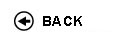Politecnico di Torino
Academic Year 2009/10
03BOQDR, 03BOQAX, 03BOQJA
Mechanics
1st degree and Bachelor-level of the Bologna process in Mechanical Engineering - Vercelli
1st degree and Bachelor-level of the Bologna process in Civil Engineering - Vercelli
1st degree and Bachelor-level of the Bologna process in Electronic And Computer Engineering - Vercelli
 Teacher Status SSD Les Ex Lab Tut Years teaching Agnello MichelangeloPO FIS/01 50 0 0 15 11
 SSD CFU Activities Area context FIS/01 5 A - Di base Fisica e chimica
 Objectives of the course The course is articulated substantially in three parts: kinematics, statics and dynamics with an introduction to the gravitational theory (force and field). In the first part the students will come supplied the physical base methods for the interpretation and the treatment of the phenomena involving vector forces and fields. The interaction between punctiform or extensive bodies, will be studied in kinematics, statics and dynamics. Particular attention will be put to the energy conservation laws. Expected skills The supplied methodologies will allow the student to deal with problems of mechanics of the punctiform body and the rigid systems. He will be able to estimate the forces that act on these bodies determining the equilibrium or the motion conditions. Moreover he will be able to estimate the energy spold from the forces that work on the bodies, included the gravitational ones, and to distinguish between the conservative forces, that accumulate the energy in the system, and the nonconservative ones that instead disperse it. Prerequisites It is necessary to know and to be able to apply base mathematics as trigonometry, vectors and vector algebra, derivatives, differential and integral calculus including line, surface and volume integrals. To the students it is strongly advised to follow the lessons and practices in classroom. Syllabus Metrology: Fundamental quantity and System of Units ' Measurements, estimations of precision and reliability of the results. Kinematics of material point: velocity and acceleration in rectilinear, simple harmonic and curvilinear motion ' Angular velocity and acceleration 'Polar, cylindrical, tangential and normal component of velocity and acceleration ' Circular motion ' General curvilinear motion ' Not relativistic relative motion ' Statics: forces, composition of forces, polar and axial torque, definition and composition ' Law of gravity, static and dynamic friction force, elastic force, wire tension ' Barycentre and center of mass ' Degree of freedom for material point ' Constraint forces ' Equilibrium of a particle and a rigid body ' Dynamics of material points: Mass concept, the law of inertia, linear momentum ' Principle of momentum conservation, Newton's second and third laws ' Equation of motion ' Initial conditions ' Motion in the given force field ' Inertial and non inertial reference system ' Non inertial forces ' Angular momentum ' Work and Energy ' Units of work and power ' Work of a force ' Kinetic energy and theorem of kinetic energy ' Conservative and nonconservative forces ' Potential energy and energy conservation laws. Dynamics of a system of particles. Motion of the center of mass ' Angular momentum of a system of particles. Kinetics energy of a system of particles ' Conservation of energy of a system of particles ' Collisions ' Fluid motion. Dynamics of a rigid body. Angular momentum of a rigid body ' alculation of the moment of inertia ' Equation of motion for rotation of a rigid body ' Kinetic energy of rotation. Laboratories and/or exercises The practices have the scope to give an help for the understanding of the theory and to show the applications of the concepts explained during the lessons. All the arguments explained in the theory are deal to you with attention. Bibliography Halliday, Resnick, Walker: Fundamentals of Physics, 7th Edition. Chapter 1-20; Mechanics/Thermodynamics. Editor: John Wiley & Sons Inc. Richard Phillips Feyman: Feyman Lectures on Physics, Editor: Leighton ' Sands Revisions / Exam The exam consists of two parts. The first one is a written test with an exercise to solve. The second one is the theoretical part, constituted from one series of quizzes. The marks obtained in each test, higher than the corresponded threshold, will be appropriately mediated in order to get the final grade. Programma definitivo per l'A.A.2009/10© Politecnico di Torino
Corso Duca degli Abruzzi, 24 - 10129 Torino, ITALY